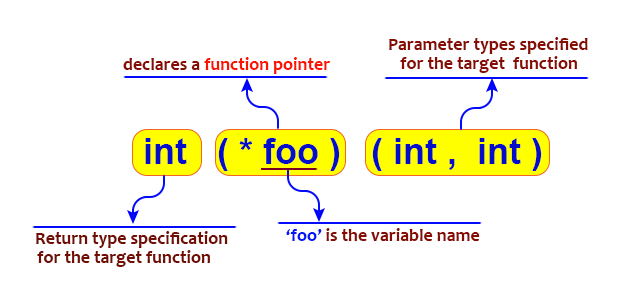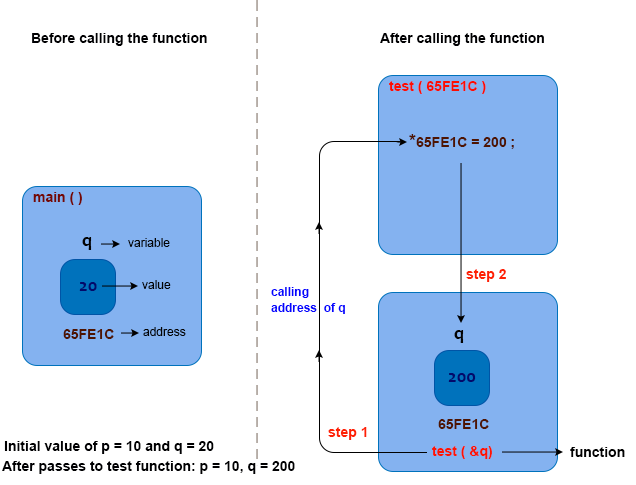# C - Pointers and functions

## Pointers and functions

The C language makes extensive use of pointers, as we have seen. They can be used to provide indirect references to primitive types, to create dynamically sized arrays, to create instances of structs on demand, and to manipulate string data, among other things. Pointers can also be used to create references to functions. In other words, a function pointer is a variable that contains the address of a function.Functions as pointers

• Memory stores the function code
• The address or start of a function is referred to as a "function pointer"
• Since function pointers do not allocate or deallocate memory, they are "different" from other pointers
• It is possible to pass function pointers as arguments to other functions or as returns from other functions

Why use function pointers?

• Efficiency
• Elegance
• Runtime binding

Function pointer declarations

Unlike a function declaration, a function pointer declaration wraps the function name in parentheses and precedes it with an asterisk. Here is an example:

• int function(int x, int y); /* a function taking two int arguments and returning an int */
• int (*pointer)(int x, int y); /* a pointer to such a function */

Pointers as Arguments

By passing a pointer into a function, the function may read or change memory outside of its activation record.

Example: Pointers and functions

In the following example, function passes the value of p and the address of q, which are used to initialize the variables x and ptry in the function test.

Code:

``````#include <stdio.h>

void test( int x, int *ptry ) {
x = 200;
*ptry = 200;
return;
}
int main( void ) {
int p = 10, q = 20;
printf( "Initial value of p = %d and q = %d\n", p, q );
test( p, &q );
printf( "After passes to test function: p = %d, q = %d\n", p, q );
return 0;
}
``````

In the said code ‘p’ remains at 10 as there is no return value form the function test, but q change its value from 20 to 200 in the function test() as bptr holds the address of a variable q that is stored in main function. So when we change the value of *bptr it will automatically reflecte in the main function.

Output:

```Initial value of p = 10 and q = 20
After passes to test function: p = 10, q = 200
```Example: Swapping two values using pointers and functions

Code:

``````#include<stdio.h>
void swap (int *p, int *q) {
int temp_val;
temp_val = *p;
*p = *q;
*q = temp_val;
}

int main()
{
int x = 45;
int y = 65;
printf( "Initial value of x = %d and y = %d\n", x, y );
swap(&x, &y);
printf( "After swapping said values x = %d and y = %d\n", x, y );
return 0;
}
``````

Output:

```Initial value of x = 45 and y = 65
After swapping said values x = 65 and y = 45
```

Pointer as Return Value

• A pointer can also be returned by functions
• In this case, the function returns the memory address of where the value is stored rather than the value itself
• Ensure that you do not return an address to a temporary variable in a function!

Example of Returning a Pointer:

Code: Maximum of two numbers

``````#include <stdio.h>

int* max(int *a, int *b) {
if (*a > *b)
return a;
return b;
}

int main() {
int x = 105, y = 102, *ptr;
printf("Original Numbers: x = %d, y = %d",x , y);
ptr = max(&x, &y);
printf("\nMaximum of said two numbers: %d", *ptr);
return 0;
}
``````

Output:

```Original Numbers: x = 105, y = 102
Maximum of said two numbers: 105
```

Previous: C Pointers
Next: C Arrays and Pointers

﻿